The GLIMMIX Procedure
 Exploring and Comparing Covariance Matrices

If you use an empirical (sandwich) estimator with the EMPIRICAL= option in the PROC GLIMMIX statement, the procedure replaces the model-based estimator of the covariance of the fixed effects with the sandwich estimator. This affects aspects of inference, such as prediction standard errors, tests of fixed effects, estimates, contrasts, and so forth. Similarly, if you choose the DDFM=KENWARDROGER degrees-of-freedom method in the MODEL statement, PROC GLIMMIX adjusts the model-based covariance matrix of the fixed effects according to Kenward and Roger (1997) or according to Kackar and Harville (1984) and Harville and Jeske (1992).

In this situation, the COVB(DETAILS) option in the MODEL statement has two effects. The GLIMMIX procedure displays the (adjusted) covariance matrix of the fixed effects and the model-based covariance matrix (for ODS purposes, the name of the table with the model-based covariance matrix is "CovBModelBased"). The procedure also displays a table of statistics for the unadjusted and adjusted covariance matrix and for their comparison. For ODS purposes, the name of this table is "CovBDetails."

If the model-based covariance matrix is not replaced with an adjusted estimator, the COVB(DETAILS) option displays the model-based covariance matrix and provides diagnostic measures for it in the "CovBDetails" table.

The table generated by the COVB(DETAILS) option consists of several sections. See Example 38.8 for an application.

The trace and log determinant of covariance matrices are general scalar summaries that are sometimes used in direct comparisons, or in formulating other statistics, such as the difference of log determinants. The trace simply represents the sum of the variances of all fixed-effects parameters. If a matrix is indefinite, the determinant is reported instead of the log determinant.

The model-based and adjusted covariance matrices should have the same general makeup of eigenvalues. There should not be any negative eigenvalues, and they should have the same numbers of positive and zero eigenvalues. A reduction in rank due to the adjustment is troublesome for aspects of inference. Negative eigenvalues are listed in the table only if they occur, because a covariance matrix should be at least positive semi-definite. However, the GLIMMIX procedure examines the model-based and adjusted covariance matrix for negative eigenvalues. The condition numbers reported by PROC GLIMMIX for positive (semi-)definite matrices are computed as the ratio of the largest and smallest nonzero eigenvalue. A large condition number reflects poor conditioning of the matrix.

Matrix norms are extensions of the concept of vector norms to measure the "length" of a matrix. The Frobenius norm of an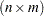matrix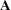is the direct equivalent of the Euclidean vector norm, the square root of the sum of the squared elements,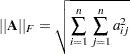The- and 1-norms of matrixare the maximum absolute row and column sums, respectively: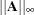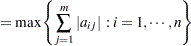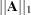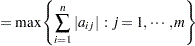These two norms are identical for symmetric matrices.

The "Comparison" section of the "CovBDetails" table provides several statistics that set the matrices in relationship. The concordance correlation reported by the GLIMMIX procedure is a standardized measure of the closeness of the model-based and adjusted covariance matrix. It is a slight modification of the covariance concordance correlation in Vonesh, Chinchilli, and Pu (1996) and Vonesh and Chinchilli (1997, Ch. 8.3). Denote as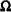the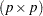model-based covariance matrix and as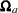the adjusted matrix. Suppose that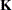is the matrix obtained from the identity matrix of sizeby replacing diagonal elements corresponding to singular rows inwith zeros. The lower triangular portion of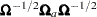is stored in vector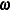and the lower triangular portion of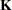is stored in vector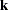. The matrix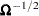is constructed from an eigenanalysis ofand is symmetric. The covariance concordance correlation is then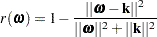This measure is 1 if=. Ifis orthogonal to, there is total disagreement between the model-based and the adjusted covariance matrix and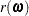is zero.

The discrepancy function reported by PROC GLIMMIX is computed as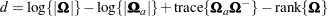In diagnosing departures between an assumed covariance structure and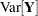—using an empirical estimator—Vonesh, Chinchilli, and Pu (1996) find that the concordance correlation is useful in detecting gross departures and propose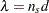to test the correctness of the assumed model, where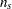denotes the number of subjects.Previous Page | Next Page | Top of Page